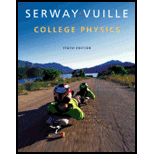# A 55-kg student eats a 540-Calorie (540 kcal) jelly doughnut for breakfast. (a) How many joules of energy are the equivalent of one jelly doughnut? (b) How many stairs must the student climb to perform an amount of mechanical work equivalent to the food energy in one jelly doughnut? Assume the height of a single stair is 15 cm. (c) If the human body is only 25% efficient in converting chemical energy to mechanical energy, how many stairs must the woman climb to work off her breakfast?### College Physics

10th Edition
Raymond A. Serway + 1 other
Publisher: Cengage Learning
ISBN: 9781285737027

#### Solutions

Chapter
Section### College Physics

10th Edition
Raymond A. Serway + 1 other
Publisher: Cengage Learning
ISBN: 9781285737027
Chapter 11, Problem 6P
Textbook Problem
17 views

## A 55-kg student eats a 540-Calorie (540 kcal) jelly doughnut for breakfast. (a) How many joules of energy are the equivalent of one jelly doughnut? (b) How many stairs must the student climb to perform an amount of mechanical work equivalent to the food energy in one jelly doughnut? Assume the height of a single stair is 15 cm. (c) If the human body is only 25% efficient in converting chemical energy to mechanical energy, how many stairs must the woman climb to work off her breakfast?

(a)

To determine
The amount of joules of energy that are equivalent of one jelly doughnut.

### Explanation of Solution

Given Info: mass of the student is 75 kg and eats 540 Calorie jelly doughnut.

Convert Calorie to Joule.

Q=540Cal(4186J1Cal)=2

(b)

To determine
The number of stairs climbed to convert the food energy to equivalent mechanical energy.

(c)

To determine
The number of stairs climbed to convert the food energy to equivalent mechanical energy if the body is 25% efficient.

### Still sussing out bartleby?

Check out a sample textbook solution.

See a sample solution

#### The Solution to Your Study Problems

Bartleby provides explanations to thousands of textbook problems written by our experts, many with advanced degrees!

Get Started

Find more solutions based on key concepts
According to genomic researchers, a single inherited gene is the probable cause of common obesity. T F

Nutrition: Concepts and Controversies - Standalone book (MindTap Course List)

Calculate the mass of 500. atoms of iron (Fe).

Chemistry: An Atoms First Approach

The tub of a washer goes into its spin cycle, starting from rest and gaining angular speed steadily for 8.00 s,...

Physics for Scientists and Engineers, Technology Update (No access codes included)

How would this unusual reproductive behavior benefit these small fish?

Oceanography: An Invitation To Marine Science, Loose-leaf Versin